## 4.3 The First Derivative Test

### Learning Objectives

• Explain how the sign of the first derivative affects the shape of a function’s graph.
• State the first derivative test for critical points.
• Find local extrema using the first derivative test.

Earlier in this chapter we stated that if a function $f$ has a local extremum at a point $c$, then $c$ must be a critical point of $f$. However, a function is not guaranteed to have a local extremum at a critical point. For example, $f(x)=x^3$ has a critical point at $x=0$ since $f^{\prime}(x)=3x^2$ is zero at $x=0$, but $f$ does not have a local extremum at $x=0$. Using the results from the previous section, we are now able to determine whether a critical point of a function actually corresponds to a local extreme value. In this section, we also see how the second derivative provides information about the shape of a graph by describing whether the graph of a function curves upward or curves downward.

# The First Derivative Test

Corollary 3 of the Mean Value Theorem showed that if the derivative of a function is positive over an interval $I$ then the function is increasing over $I$. On the other hand, if the derivative of the function is negative over an interval $I$, then the function is decreasing over $I$ as shown in the following figure.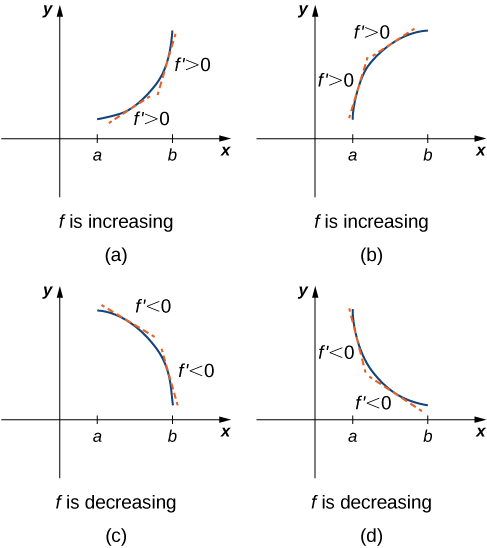Figure 1. Both functions are increasing over the interval $(a,b)$. At each point $x$, the derivative $f^{\prime}(x)>0$. Both functions are decreasing over the interval $(a,b)$. At each point $x$, the derivative $f^{\prime}(x)<0$.

A continuous function $f$ has a local maximum at point $c$ if and only if $f$ switches from increasing to decreasing at point $c$. Similarly, $f$ has a local minimum at $c$ if and only if $f$ switches from decreasing to increasing at $c$. If $f$ is a continuous function over an interval $I$ containing $c$ and differentiable over $I$, except possibly at $c$, the only way $f$ can switch from increasing to decreasing (or vice versa) at point $c$ is if ${f}^{\prime }$ changes sign as $x$ increases through $c.$ If $f$ is differentiable at $c,$ the only way that ${f}^{\prime }.$ can change sign as $x$ increases through $c$ is if $f^{\prime}(c)=0$. Therefore, for a function $f$ that is continuous over an interval $I$ containing $c$ and differentiable over $I$, except possibly at $c$, the only way $f$ can switch from increasing to decreasing (or vice versa) is if $f^{\prime}(c)=0$ or $f^{\prime}(c)$ is undefined. Consequently, to locate local extrema for a function $f$, we look for points $c$ in the domain of $f$ such that $f^{\prime}(c)=0$ or $f^{\prime}(c)$ is undefined. Recall that such points are called critical points of $f$.

Note that $f$ need not have a local extrema at a critical point. The critical points are candidates for local extrema only. In (Figure), we show that if a continuous function $f$ has a local extremum, it must occur at a critical point, but a function may not have a local extremum at a critical point. We show that if $f$ has a local extremum at a critical point, then the sign of $f^{\prime}$ switches as $x$ increases through that point.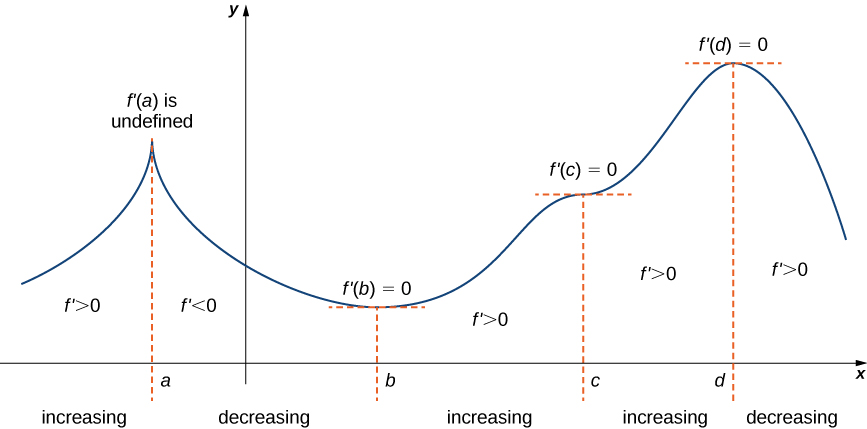Figure 2. The function $f$ has four critical points: $a,b,c$, and $d$. The function $f$ has local maxima at $a$ and $d$, and a local minimum at $b$. The function $f$ does not have a local extremum at $c$. The sign of $f^{\prime}$ changes at all local extrema.

Using (Figure), we summarize the main results regarding local extrema.

• If a continuous function $f$ has a local extremum, it must occur at a critical point $c$.
• The function has a local extremum at the critical point $c$ if and only if the derivative $f^{\prime}$ switches sign as $x$ increases through $c$.
• Therefore, to test whether a function has a local extremum at a critical point $c$, we must determine the sign of $f^{\prime}(x)$ to the left and right of $c$.

This result is known as the first derivative test.

### First Derivative Test

Suppose that $f$ is a continuous function over an interval $I$ containing a critical point $c$. If $f$ is differentiable over $I$, except possibly at point $c$, then $f(c)$ satisfies one of the following descriptions:

1. If $f^{\prime}$ changes sign from positive when $x<c$ to negative when $x>c$, then $f(c)$ is a local maximum of $f$.
2. If $f^{\prime}$ changes sign from negative when $x<c$ to positive when $x>c$, then $f(c)$ is a local minimum of $f$.
3. If $f^{\prime}$ has the same sign for $x<c$ and $x>c$, then $f(c)$ is neither a local maximum nor a local minimum of $f$.

We can summarize the first derivative test as a strategy for locating local extrema.

### Problem-Solving Strategy: Using the First Derivative Test

Consider a function $f$ that is continuous over an interval $I$.

1. Find all critical points of $f$ and divide the interval $I$ into smaller intervals using the critical points as endpoints.
2. Analyze the sign of $f^{\prime}$ in each of the subintervals. If $f^{\prime}$ is continuous over a given subinterval (which is typically the case), then the sign of $f^{\prime}$ in that subinterval does not change and, therefore, can be determined by choosing an arbitrary test point $x$ in that subinterval and by evaluating the sign of $f^{\prime}$ at that test point. Use the sign analysis to determine whether $f$ is increasing or decreasing over that interval.
3. Use (Figure) and the results of step 2 to determine whether $f$ has a local maximum, a local minimum, or neither at each of the critical points.

Now let’s look at how to use this strategy to locate all local extrema for particular functions.

### Using the First Derivative Test to Find Local Extrema

Use the first derivative test to find the location of all local extrema for $f(x)=x^3-3x^2-9x-1$. Use a graphing utility to confirm your results.

Use the first derivative test to locate all local extrema for $f(x)=−x^3+\frac{3}{2}x^2+18x$.

#### Hint

Find all critical points of $f$ and determine the signs of $f^{\prime}(x)$ over particular intervals determined by the critical points.

### Using the First Derivative Test

Use the first derivative test to find the location of all local extrema for $f(x)=5x^{1/3}-x^{5/3}$. Use a graphing utility to confirm your results.

Use the first derivative test to find all local extrema for $f(x)=\sqrt{x-1}$.

#### Hint

The only critical point of $f$ is $x=1$.

### Key Concepts

• If $c$ is a critical point of $f$ and $f^{\prime}(x)>0$ for $x<c$ and $f^{\prime}(x)<0$ for $x>c$, then $f$ has a local maximum at $c$.
• If $c$ is a critical point of $f$ and $f^{\prime}(x)<0$ for $x<c$ and $f^{\prime}(x)>0$ for $x>c$, then $f$ has a local minimum at $c$.

1. If $c$ is a critical point of $f(x)$, when is there no local maximum or minimum at $c$? Explain.

For the following exercises, analyze the graphs of $f^{\prime}$, then list all intervals where $f$ is increasing or decreasing.

2.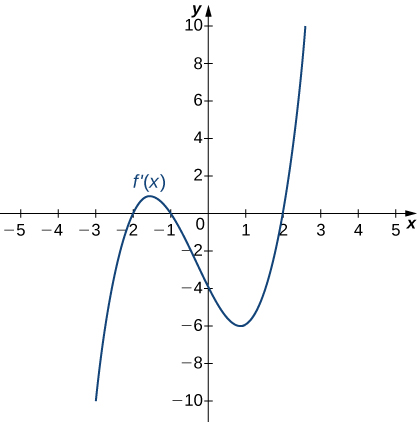3.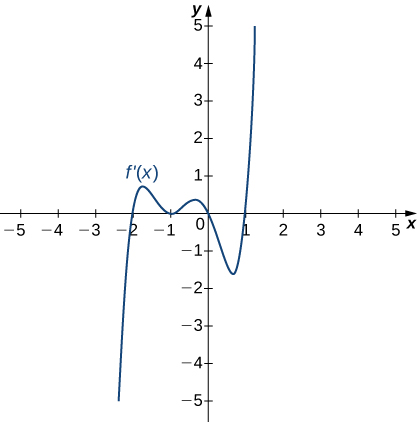4.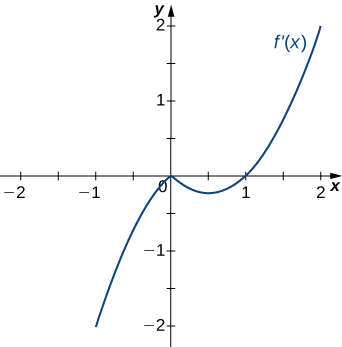5.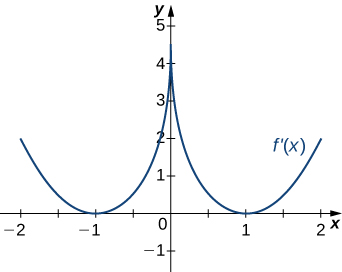6.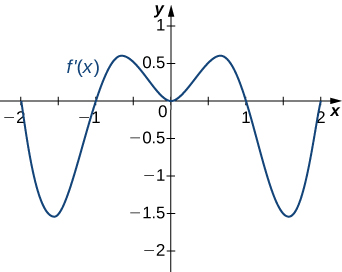For the following exercises, analyze the graphs of $f^{\prime}$, then list

1. all intervals where $f$ is increasing and decreasing and
2. where the minima and maxima are located.
7.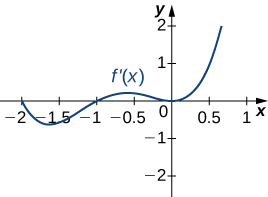8.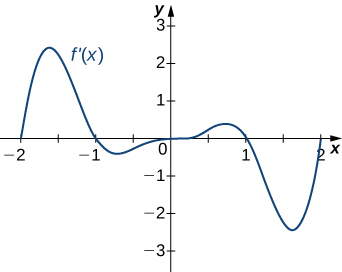9.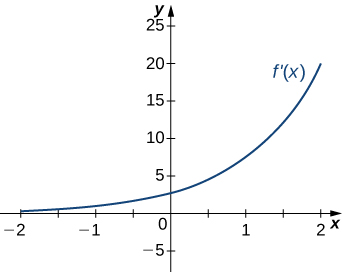10.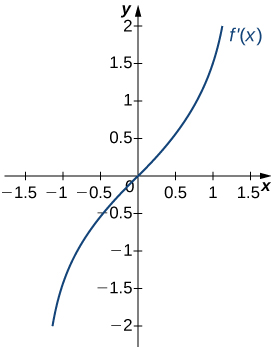11.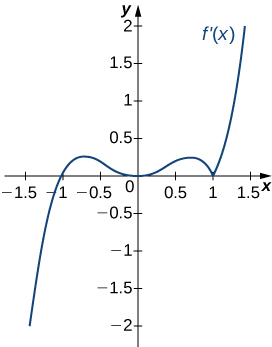For the following exercises, determine

1. intervals where $f$ is increasing or decreasing (in interval notation) and
2. local minima and maxima (as a coordinate).

12. $f(x)=-x^2-3x+3$

13. $f(x)=6x-x^3$

14. $f(x)=3x^2-4x^3$

15. $g(t)=t^4-4t^3+4t^2$

16. $f(t)=t^4-8t^2+16$

17. $h(x)=4\sqrt{x}-x^2+3$

18. $h(x)=x-6\sqrt{x-1}$

19. $g(x)=x^{2}\sqrt{5-x}$

20. $g(x)=x\sqrt{8-x^{2}}$

21. $f(\theta)=\theta^2 + \cos \theta$

22. $f(\theta)= \sin \theta+ \sin^3 \theta$ over the interval $\left(-\pi,\pi\right)$

23. $f(x)=\frac{x^3}{3x^2+1}$

24. $f(x)=\frac{x^2-3}{x-2}$

25. $h(x)=x^{\frac{2}{3}}(x+5)$

26. $h(x)=x^{\frac{1}{3}}(x+8)$

27. $f(x)=e^{\sqrt{x}}$

28. $f(x)=e^{2x}+e^{-x}$

29. $f(t)=t^{2}\ln t$

30. $f(t)=8t \ln t$

## Glossary

concave down
if $f$ is differentiable over an interval $I$ and $f^{\prime}$ is decreasing over $I$, then $f$ is concave down over $I$
concave up
if $f$ is differentiable over an interval $I$ and $f^{\prime}$ is increasing over $I$, then $f$ is concave up over $I$
concavity
the upward or downward curve of the graph of a function
concavity test
suppose $f$ is twice differentiable over an interval $I$; if $f^{\prime \prime}>0$ over $I$, then $f$ is concave up over $I$; if $f^{\prime \prime}<0$ over $I$, then $f$ is concave down over $I$
first derivative test
let $f$ be a continuous function over an interval $I$ containing a critical point $c$ such that $f$ is differentiable over $I$ except possibly at $c$; if $f^{\prime}$ changes sign from positive to negative as $x$ increases through $c$, then $f$ has a local maximum at $c$; if $f^{\prime}$ changes sign from negative to positive as $x$ increases through $c$, then $f$ has a local minimum at $c$; if $f^{\prime}$ does not change sign as $x$ increases through $c$, then $f$ does not have a local extremum at $c$
inflection point
if $f$ is continuous at $c$ and $f$ changes concavity at $c$, the point $(c,f(c))$ is an inflection point of $f$
second derivative test
suppose $f^{\prime}(c)=0$ and $f^{\prime \prime}$ is continuous over an interval containing $c$; if $f^{\prime \prime}(c)>0$, then $f$ has a local minimum at $c$; if $f^{\prime \prime}(c)<0$, then $f$ has a local maximum at $c$; if $f^{\prime \prime}(c)=0$, then the test is inconclusive